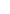## Operational amplifiers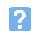1. The operational amplifier shown in the photo below is supplied in: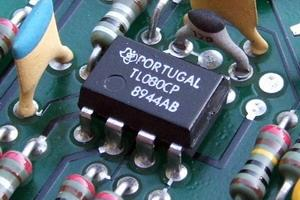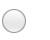a) a flatpackb) a DIL packagec) a SIL packaged) a leadless surface mounting package.2. An ideal operational amplifier would have:a) very low input impedance, very high output impedance and very low voltage gainb) very high input impedance, very low output impedance and very low voltage gainc) very high input impedance, very low output impedance and very high voltage gaind) very high input impedance, very high output impedance and very high voltage gain3. The connection marked X in the diagram shown below is: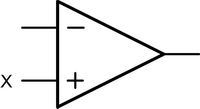a) the negative supplyb) the positive supplyc) the non-inverting inputd) the common 0V connection.4. Which one of the following is a typical value of open-loop voltage gain for an operational amplifier?a) 200b) 2000c) 20000d) 200000.5. The units of slew-rate are:a) ohms per voltb) volts per ohmc) seconds per voltd) volts per second.6. An operational amplifier has a gain x bandwidth product of 2 MHz. If the device is used as an inverting amplifier a gain of 50, which one of the following gives the resulting bandwidth?a) 10 kHzb) 40 kHzc) 100 kHzd) 400 kHz.7. In the circuit shown below, the mid-band voltage gain will be given by: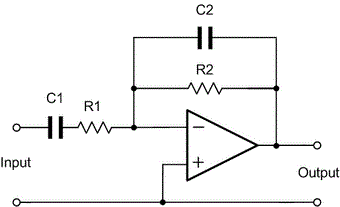a) R1 + R2b) R2 - R1c) R2 / R1d) R1 / R2.8. The typical value of output resistance for an operational amplifier is in the range:a) 1 ohm to 10 ohmb) 10 ohm to 100 ohmc) 100 ohm to 1 kohmd) 1 kohm to 10 kohm.9. Which one of the following gives the output voltage produced by the circuit shown below?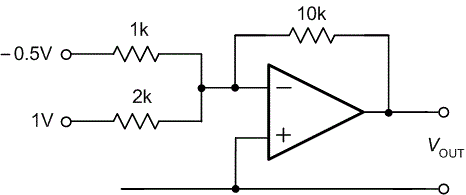a) -2.5Vb) 0Vc) 2.5Vd) 5V.10. When negative feedback is applied to an operational amplifier:a) the overall voltage gain is reduced and the bandwidth is reducedb) the overall voltage gain is increased and the bandwidth is reducedc) the overall voltage gain is reduced and the bandwidth is increasedd) the overall voltage gain is increased and the bandwidth is increased.
This is more feedback!
This is the feedback!